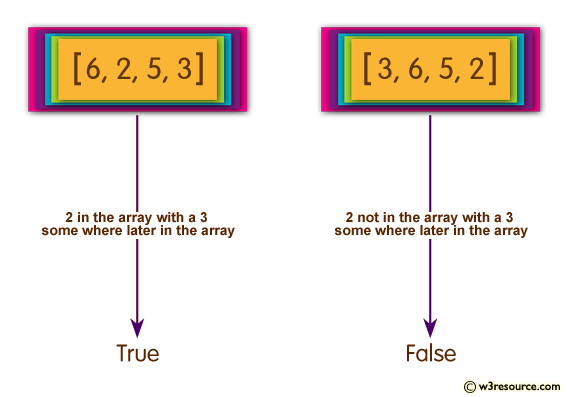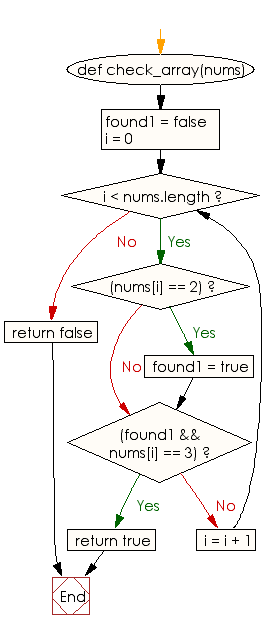﻿ Ruby Array exercises: Check whether there is a 2 in the array with a 3 some where later in a given array of integers - w3resource# Ruby Array Exercises: Check whether there is a 2 in the array with a 3 some where later in a given array of integers

## Ruby Array: Exercise-40 with Solution

Write a Ruby program to check whether there is a 2 in the array with a 3 some where later in a given array of integers.Ruby Code:

``````def check_array(nums)
found1 = false
i = 0
while i < nums.length
if(nums[i] == 2)
found1 = true
end
if(found1 && nums[i] == 3)
return true
end
i = i + 1
end
return false
end
print check_array([6, 2, 3, 5]),"\n"
print check_array([2, 6, 5, 3]),"\n"
print check_array([6, 2, 5, 3]),"\n"
print check_array([3, 6, 5, 2]),"\n"
print check_array([3, 3, 2, 1]),"\n"
``````

Output:

```true
true
true
false
false
```

Flowchart:Ruby Code Editor: